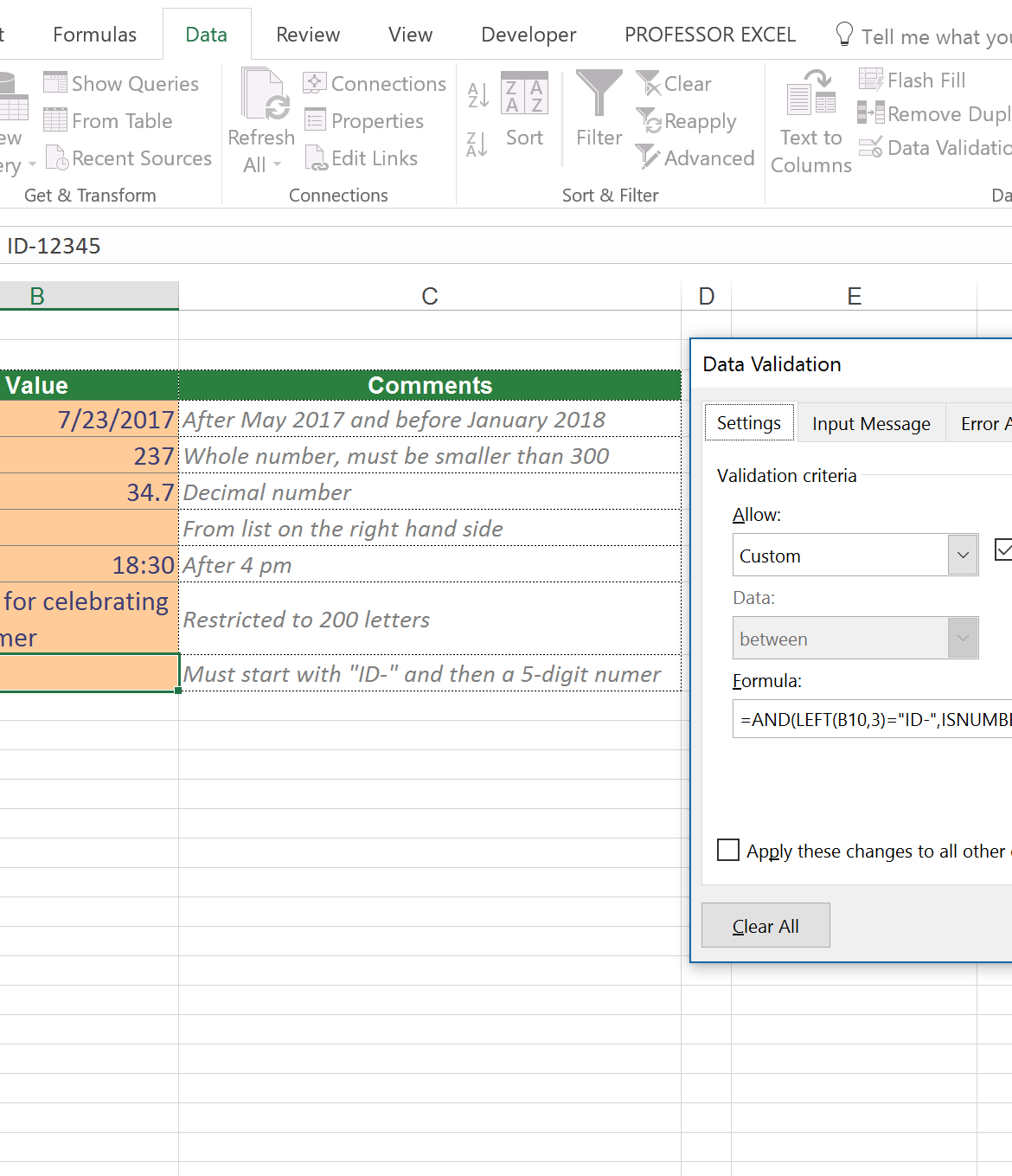##### Published September 11, 2016 at 2171 × 1359 in Data Validation in Excel: Restrict Cells to Allow Only Certain Values.7) The most complicated option: Use a formula to restrict input values. In this case the input value must start with “ID-” and must be followed by a 5-digit number. The complete formula is: =AND(LEFT(B10,3)=”ID-“,ISNUMBER(RIGHT(B10,5)*1))